Courses

Test: Syllogism- 1

15 Questions MCQ Test Logical Reasoning for CLAT | Test: Syllogism- 1

Description
Attempt Test: Syllogism- 1 | 15 questions in 25 minutes | Mock test for CLAT preparation | Free important questions MCQ to study Logical Reasoning for CLAT for CLAT Exam | Download free PDF with solutions
QUESTION: 1

In each of the questions below given four statements followed by three conclusions numbered I, II and III. You have to take the given statements to be true even if they seem to be at variance from commonly known facts. Read all the conclusions and then decide which of the given conclusions logically follows from the given statements disregarding commonly known facts.​ Q. Statements: All cards are notes. All notes are tyres. All tyres are books. All books are rods. Conclusions: I. Some rods are notes. II. Some books are tyres. III. Some tyres are cards

Solution: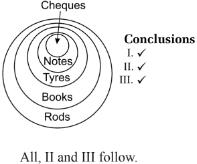QUESTION: 2

In each of the questions below given four statements followed by three conclusions numbered I, II and III. You have to take the given statements to be true even if they seem to be at variance from commonly known facts. Read all the conclusions and then decide which of the given conclusions logically follows from the given statements disregarding commonly known facts. ​Q. ​ ​Statements: Some seeds are flowers. All flowers are trees. All trees are leaves. Some leaves are plants Conclusions: I. Some plants are flowers. II. Some leaves are seeds. III. Some leaves are flowers.

Solution:

It is clear from the venn diagram that only conclusion 2 and 3 follows.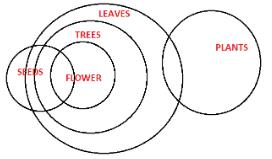QUESTION: 3

In each of the questions below given four statements followed by three conclusions numbered I, II and III. You have to take the given statements to be true even if they seem to be at variance from commonly known facts. Read all the conclusions and then decide which of the given conclusions logically follows from the given statements disregarding commonly known facts. ​Q. ​​ Statements: Some lenses are poles. All poles are skies. Some skies are boxes. No box is a bottle. Conclusions: I. Some bottles are lens II. Some boxes are poles. III. No bottle is a lens.

Solution:
QUESTION: 4

In the questions below are given four statements followed by for conclusions numbered I, II, III and IV. You have to take given statements to be true even if they seem to be at variance from commonly known facts. Read all the conclusions and then decide which of the given conclusions logically follows from the given statements disregarding commonly known facts.

Q.
Statements:
All cups are bottles.
Some bottles are mugs.
No mug is a plate.
Some plates are tables.

Conclusions:

I. Some tables are bottles.

II. Some plates are cups

III. No table is a bottle.

IV. Some mugs are cups.

Solution:

The Venn diagram for the syllogism is as shown below: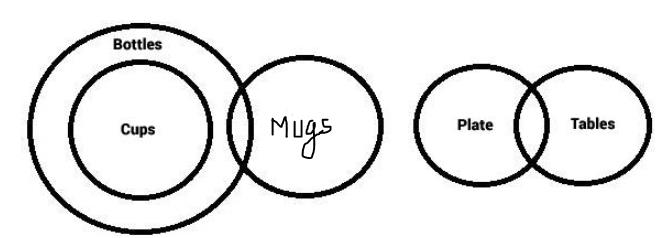The conclusions I and III are complementary in nature ie. One of the two has to be correct universally and both can't be correct at the same time. And the other conclusions are false. Hence, option (E) is the right answer.

QUESTION: 5

In the questions below are given four statements followed by for conclusions numbered I, II, III and IV. You have to take given statements to be true even if they seem to be at variance from commonly known facts. Read all the conclusions and then decide which of the given conclusions logically follows from the given statements disregarding commonly known facts.

Q.​
Statements:
All birds are horses.
All horses are tigers.
Some tigers are lions.
Some lions are monkeys.

Conclusions:

I. Some tigers are horses.

II. Some monkeys are birds.

III. Some tigers are birds.

IV. Some monkeys are horses.

Solution: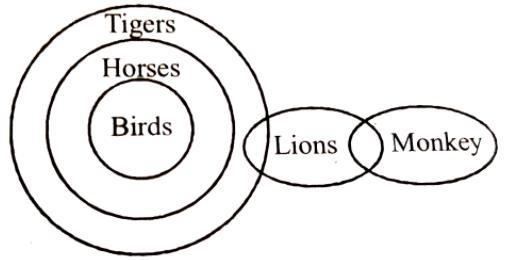Conclusions:
I. True
II. False
III. True
IV. False
So, only conclusion I & III follow

QUESTION: 6

In the questions below are given four statements followed by for conclusions numbered I, II, III and IV. You have to the take given statements to be true even if they seem to be at variance from commonly known facts. Read all the conclusions and then decide which of the given conclusion logically follows from the given statements disregarding commonly known facts.

Q.​ ​​Statements:
Some Clothes are lamps.
Some flowers are lamps.
Some lamps are dresses.
All dresses are shirts.

Conclusions:

I. Some shirts are Clothes

II. Some shirts are flowers.

III. Some flowers are Clothes.

IV. Some dresses are Clothes.

Solution:

A is the correct option
lamps = some clothes, some flowers and some dresses.
All dresses are shirts, meaning, some lamps are shirts.

Hence, none of the conclusion is correct.

QUESTION: 7

In each of the questions below are given four statements followed by for conclusions numbered I, II, III and IV. You have to the take given statements to be true even if they seem to be at variance from commonly known facts. Read all the conclusions and then decide which of the given conclusion logically follows from the given statements disregarding commonly known facts.

Q.​ ​​​
Statements:
Some benches are walls.
All walls are houses.
Some houses are jungles.
All jungles are windows.

Conclusions:

I. Some windows are benches.

II. Some jungles are walls.

III. Some houses are benches.

IV. Some windows are houses.

Solution:

The correct option is C.
The venn diagram for the question is follow-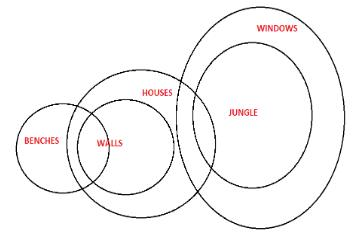QUESTION: 8

In each of the questions below are given four statements followed by for conclusions numbered I, II, III and IV. You have to take given statements to be true even if they seem to be at variance from commonly known facts. Read all the conclusions and then decide which of the given conclusion logically follows from the given statements disregarding commonly known facts.
Q.
Statements :

No cream is a lemon.

All lemons are onions.

All cream is a grapes.

Conclusion :

(i) Some grapes are not onions.

(ii) All lemons are grapes is a possibility

Solution:
QUESTION: 9

In each of the questions below are given four statements followed by for conclusions numbered I, II, III and IV. You have to the take given statements to be true even if they seem to be at variance from commonly known facts. Read all the conclusions and then decide which of the given conclusion logically follows from the given statements disregarding commonly known facts.
Q.
Statements :

Some trees are mangoes.

All mangoes are bananas.

All tomatoes are bananas.

Conclusions:

(i) All trees are tomatoes is a possibility.

(ii) No bananas are tree is a possibility.

Solution:

The answer is A because some mangoes are trees and all mangoes are bananas so some mangoes which are tree would come into bananas always hence conclusion II is not implicit.

QUESTION: 10

In each of the questions below are given four statements followed by for conclusions numbered I, II, III and IV. You have to the take given statements to be true even if they seem to be at variance from commonly known facts. Read all the conclusions and then decide which of the given conclusion logically follows from the given statements disregarding commonly known facts.
Q.
Statements :

All mobiles are cellular.

All cellular are mechanics.

All microphones are mobiles.

Conclusions :

(i) No microphone is mechanic is a possibility.

(ii) Some microphones are not cellular.

Solution:

The correct option is D.
From the venn diagram it is clear that
all microphones are possible to be mechanic and Some microphones are cellular.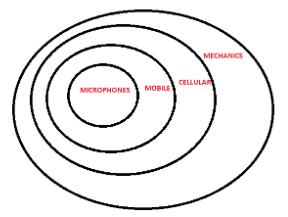QUESTION: 11

In each of the questions below are given four statements followed by for conclusions numbered I, II, III and IV. You have to the take given statements to be true even if they seem to be at variance from commonly known facts. Read all the conclusions and then decide which of the given conclusion logically follows from the given statements disregarding commonly known facts.
Q.
Statements :

All mobiles are cellular.

All cellular are mechanics.

All microphones are mobiles.

Conclusions :

(i) Some mechanics which one mobiles are also a part of microphones.

(ii) All microphones are cellular.

Solution:

The correct option is E.
It is clear from the venn diagram.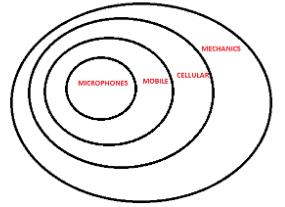QUESTION: 12

In each of the questions below are given four statements followed by for conclusions numbered I, II, III and IV. You have to the take given statements to be true even if they seem to be at variance from commonly known facts. Read all the conclusions and then decide which of the given conclusion logically follows from the given statements disregarding commonly known facts.
Q.
Statements :

All pencils are pens.

Some pens are stickers.

All stickers are numbers.

Some number are erasers.

Conclusions :

(i) Some numbers are pens.

(ii) Some numbers are stickers.

Solution: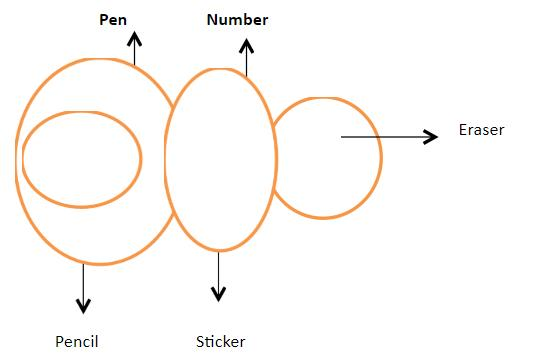QUESTION: 13

In each of the questions below are given four statements followed by for conclusions numbered I, II, III and IV. You have to the take given statements to be true even if they seem to be at variance from commonly known facts. Read all the conclusions and then decide which of the given conclusion logically follows from the given statements disregarding commonly known facts.
Q.
Statements :

All dusters are bowls.

All bowls are shoes.

No shoe is a root.

All roots are flowers.

Conclusions :

(i) No flower is a bowl.

(ii) All dusters are shoes.

Solution:

It is clear from the venn diagram that both the conclusions follow.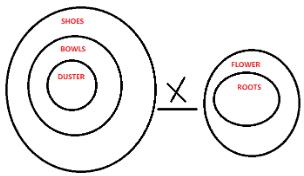QUESTION: 14

In each of the questions below are given four statements followed by for conclusions numbered I, II, III and IV. You have to the take given statements to be true even if they seem to be at variance from commonly known facts. Read all the conclusions and then decide which of the given conclusion logically follows from the given statements disregarding commonly known facts.
Q.
Statements :

All dusters are bowls.

All bowls are shoes.

No shoe is a root.

All roots are flowers.

Conclusions :

(i) No duster is a root.

(ii) All flowers are root.

Solution:

The correct option is A.
As if all roots are flowers that do not means that all flowers are roots, thus only conclusion 1 follows.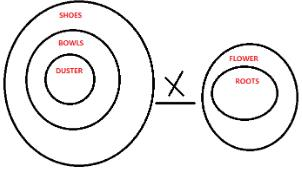QUESTION: 15

In each of the questions below are given four statements followed by for conclusions numbered I, II, III and IV. You have to the take given statements to be true even if they seem to be at variance from commonly known facts. Read all the conclusions and then decide which of the given conclusion logically follows from the given statements disregarding commonly known facts.
Q.
Statements :

Some mails are chats.

Conclusions :

(i) All mails being uploads is a possibility.

(ii) No upload is a mail.

Solution:

Some mails are chats. There is a possibility that all mails are chats. All updates are chats. So, if mails is a subset of updates, which in turn is a subset of chats, the conditions still hold true. So, conclusion I follows. Conclusion II is not necessarily true as there might be some intersection between mails and updates.Use Code STAYHOME200 and get INR 200 additional OFF Use Coupon Code

Track your progress, build streaks, highlight & save important lessons and more!

Similar Content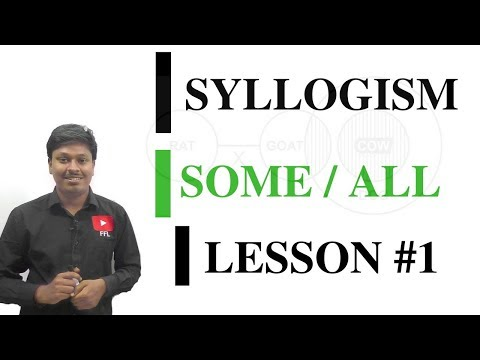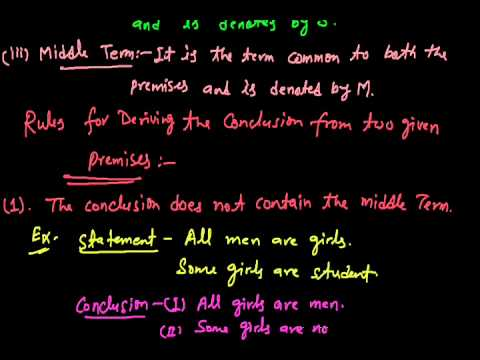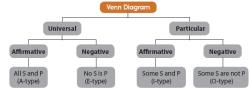Related tests